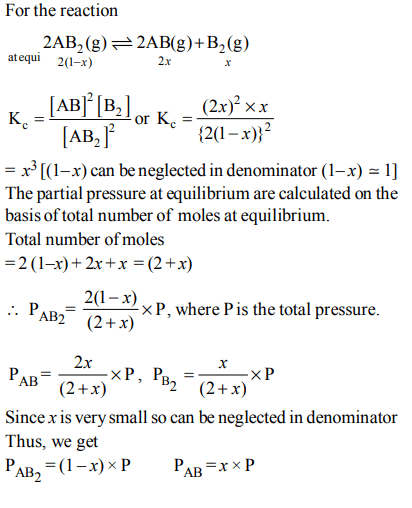## Chemical Equilibrium Questions and Answers Part-8

1. The partial pressure of $CH_{3}OH\left(g\right), CO\left(g\right) and H_{2}\left(g\right)$       in equilibrium mixture for the reaction,
$CO\left(g\right) + 2H_{2}\left(g\right)\rightleftharpoons CH_{3} OH\left(g\right)$      are 2.0, 1.0 and 0.1 atm respectively at 427°C. The value of $K_{P}$ for the decomposition of $CH_{3}OH$   to CO and $H_{2}$   is
a) $10^{2}atm$
b) $2 × 10^{2}atm^{-1}$
c) $50 atm^{2}$
d) $5 × 10^{-3}atm^{2}$

Explanation: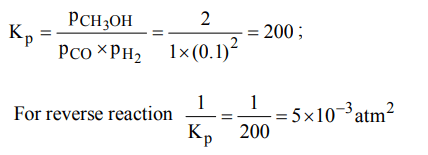2. Which of the following is not affected by pressure change?
a) $2NO_{2}\left(g\right)\rightleftharpoons N_{2}O_{4}\left(g\right)$
b) $CO_{2}\left(g\right)+H_{2}O\left(l\right)\rightleftharpoons CO_{2} solution$
c) $H_{2}\left(g\right)+I_{2}\left(g\right)\rightleftharpoons 2HI\left(g\right)$
d) $2O_{3}\left(g\right)\rightleftharpoons 3O_{2}\left(g\right)$

Explanation: No effect of pressure when $\triangle$n = 0.

3. The degree of dissociation of dinitrogen tetroxide $N_{2}O_{4}\left(g\right)\rightarrow 2NO_{2}\left(g\right)$     at temperature T and total pressure P is $\alpha$ . Which one of the following is the correct expression for the equilibrium constant $\left(K_{P}\right)$ at this temperature?
a) $\frac{2\alpha}{\left(1-\alpha^{2}\right)}$
b) $\frac{\alpha^{2}P}{1-\alpha}$
c) $\frac{4\alpha^{2}}{\left(1-\alpha ^{2}\right)}$
d) $\frac{4\alpha^{2}P}{\left(1-\alpha ^{2}\right)}$

Explanation:4. Calculate the degree of dissociation of $PCl_{5}$  , the density at 230°C is 70
a) 97.8%
b) 48.9%
c) 4.89%
d) 24.45%

Explanation: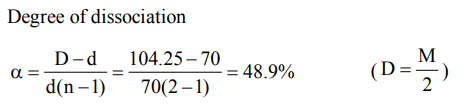5. When heated to 100°C the V.D. of $N_{2}O_{4}$ becomes 24.5. The degree of dissociation of $N_{2}O_{4}$ at this temp. will be
a) 80%
b) 87.74%
c) 40.34%
d) 60%

Explanation: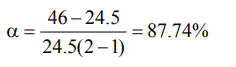6. The correct relationship between free energy change in a reaction and the corresponding equilibrium constant $K_{C}$ is
a) $\triangle G=RT ln K_{C}$
b) $-\triangle G=RT ln K_{C}$
c) $\triangle G^{\circ}=RT ln K_{C}$
d) $-\triangle G^{\circ}=RT ln K_{C}$

Explanation: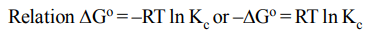7. The reaction quotient (Q) for the reaction $N_{2}\left(g\right)+3H_{2}\left(g\right)\rightleftharpoons 2NH_{3}\left(g\right)$
is given by $Q=\frac{\left[NH_{3}\right]^{2}}{\left[N_{2}\right]\left[H_{2}\right]^{3}}$    . The reaction will proceed from right to left if
a) $Q<K_{C}$
b) $Q>K_{C}$
c) Q=0
d) $Q=K_{C}$

Explanation: Q > Kc will make the reaction to proceed from right to left.

8. In Haber process 30 litres of dihydrogen and 30 litres of dinitrogen were taken for reaction which yielded only 50% of the expected product. What will be the composition of gaseous mixture under the aforesaid conditions in the end?
a) 10 litres of ammonia, 25 litres nitrogen, 15 litres hydrogen
b) 20 litres ammonia, 10 litres nitrogen, 30 litres hydrogen
c) 20 litres ammonia, 25 litres nitrogen, 15 litres hydrogen
d) 20 litres ammonia, 20 litres nitrogen, 20 litres hydrogen

Explanation:9. The following equilibrium constants are given:
$N_{2}+3H_{2}\rightleftharpoons 2NH_{3} ;K_{1}$
$N_{2}+O_{2}\rightleftharpoons 2NO ;K_{2}$
$H_{2}+\frac{1}{2}O_{2}\rightleftharpoons H_{2}O;K_{3}$
The equilibrium constant for the oxidation of $NH_{3}$ by oxygen to give NO is
a) $\frac{K_{2}K_3^2}{K_{1}}$
b) $\frac{K_3^2K_{3}}{K_{1}}$
c) $\frac{K_{1}K_{2}}{K_{3}}$
d) $\frac{K_{2}K_3^3}{K_{1}}$

Explanation:10. The dissociation equilibrium of a gas $AB_{2}$ can be represented as :
$2AB_{2}\left(g\right)\rightleftharpoons 2AB\left(g\right)+B_{2}\left(g\right)$
The degree of dissociation is ‘x’ and is small compared to 1. The expression relating the degree of dissociation (x) with equilibrium constant $K_{P}$ and total pressure P is :
a) $\left(2K_{P}/P\right)$
b) $\left(2K_{P}/P\right)^{1/3}$
c) $\left(2K_{P}/P\right)^{1/2}$
d) $\left(K_{P}/P\right)$